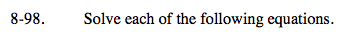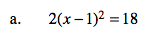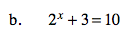Home > A2C > Chapter 8 > Lesson 8.1.6 > Problem8-98

8-98.
1. Solve each of the following equations. Homework Help ✎

1. 2(x − 1)2 = 18

2. 2x + 3 = 10Divide both sides by 2.
How many solutions are you expecting?Subtract 3 from both sides.

2x = 7

Take the log of both sides.

log (2)x = log(7)

Apply the Power Property.

x · log(2) = log(7)